SSC BOARD PAPERS IMPORTANT TOPICS COVERED FOR BOARD EXAM 2024

### Practice Set 7.2 Mensuration Class 10th Mathematics Part 2 MHB Solution

Practice Set 7.2

1. The radii of two circular ends of frustum shape bucket are 14 cm and 7 cm. Height of…
2. The radii of ends of a frustum are 14 cm and 6 cm respectively and its height is 6 cm.…
3. The circumferences of circular faces of a frustum are 132 cm and 88 cm and its height…

###### Practice Set 7.2

Question 1.

The radii of two circular ends of frustum shape bucket are 14 cm and 7 cm. Height of the bucket is 30 cm. How many liters of water it can hold? (1 litre = 1000cm3)

The two radii of frustum are, r1 = 14cm and r2 = 7cm

Height of bucket, H = 30 cm

As we know that,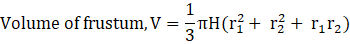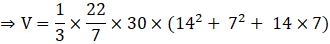⇒ V = 10780 cm3

Now, as 1litre = 1000cm3

⇒ V = 10.780 litre

∴ Bucket can hold 10.780 litres of water

Question 2.

The radii of ends of a frustum are 14 cm and 6 cm respectively and its height is 6 cm. Find its

i) Curved surface area

ii) Total surface area

iii) Volume

(i) The two radii of frustum are, r1 = 14cm and r2= 6cm

Height of frustum, H = 6cm

Slant height of frustum,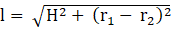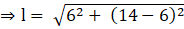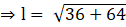⇒ l = 10

As we know that,

Curved surface area, AC = πl(r1 + r2)

⇒ AC = (3.14) × 10 × (14 + 6)

⇒ AC = (3.14) × 200

⇒ AC = 628 sq. cm

∴ the curved surface area of frustum is 628 sq. cm

(ii) Total surface area, AT = Curved surface area + area of the two circular regions

AT = AC + πr12 + πr22

On substituting the above values, we get,

⇒ AT = 628 + (3.14) × (142 + 62)

⇒ AT = 628 + (3.14)× (196 + 36)

⇒ AT = 628 + 728.48

⇒ AT = 1356.48 sq. cm

∴ the total surface area of frustum is 1356.48 cm2

(iii) As we know,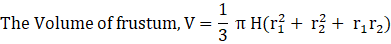On substituting the values, we get,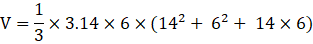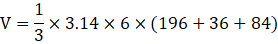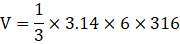V = 1984.48 cm3

∴ Volume of the frustum is 1984.48 cm3

Question 3.

The circumferences of circular faces of a frustum are 132 cm and 88 cm and its height is 24 cm. To find the curved surface area of the frustum complete the following activity.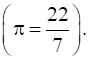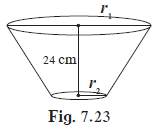Circumference1 = 2πr1 = 132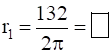Circumference2 = 2πr2 = 88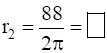Slant height of frustum,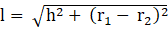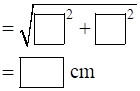Curved Surface area of frustum = π(r1 + r2)l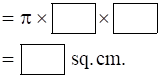Circumference1 = 2πr1 = 132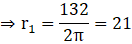Circumference2 = 2πr2 = 88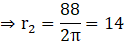Slant height of frustum,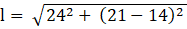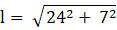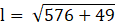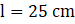Curved Surface area of frustum = π(r1 + r2)l

⇒ Curved Surface Area of frustum = π× (21 + 14) × 25

⇒ Curved surface area = π × 35 × 25 = 2750 sq. cm